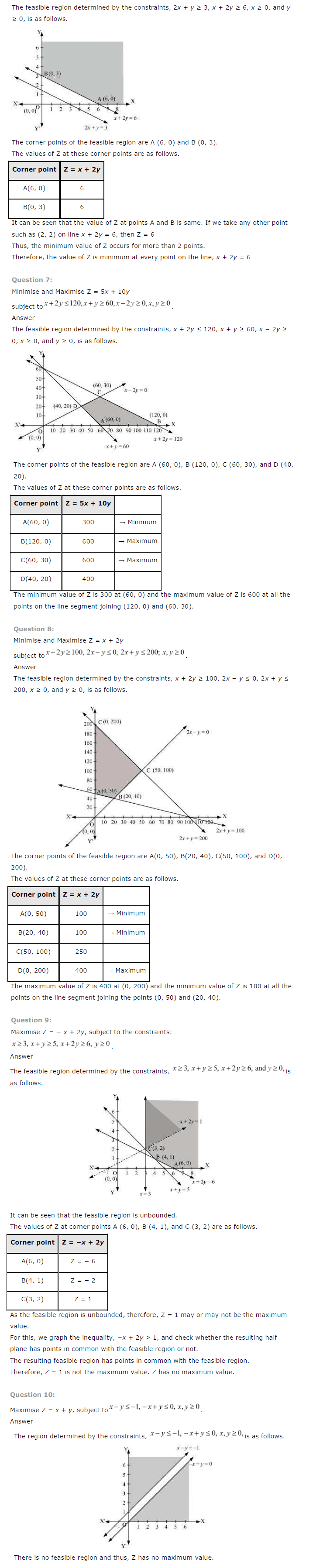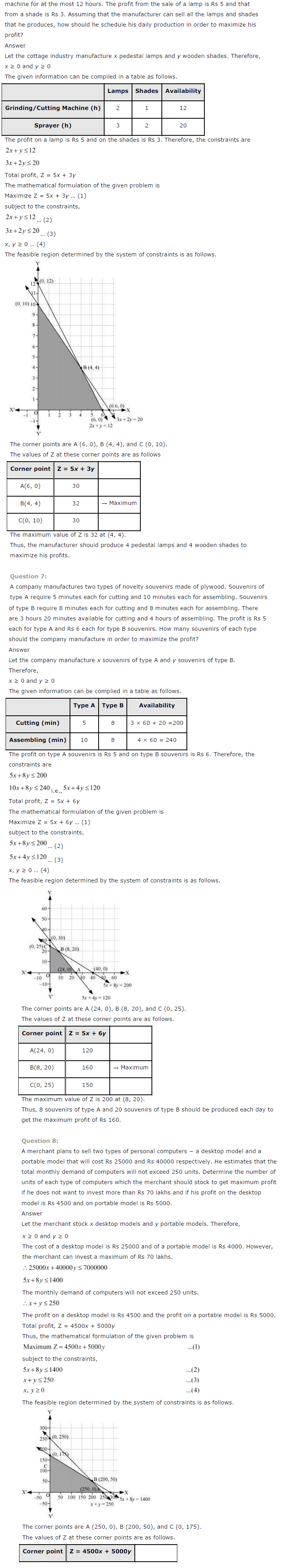NCERT Solutions for Class 12 Maths Chapter 12 Linear Programming

NCERT Solutions for Class 12 Maths Chapter 12 Linear Programming is designed and prepared by the best teachers across India. All the important topics are covered in the exercises and each answer comes with a detailed explanation to help students understand concepts better. These NCERT solutions play a crucial role in your preparation for all exams conducted by the CBSE, including the JEE.

Chapter 12 – Linear Programming covers multiple exercises. The answer to each question in every exercise is provided along with complete, step-wise solutions for your better understanding. This will prove to be most helpful to you in your home assignments as well as practice sessions.
The topics and sub-topics included in the Linear Programming chapter are the following:

 Section Name Topic Name 12 Linear Programming 12.1 Introduction 12.2 Linear Programming Problem and its Mathematical Formulation 12.3 Different Types of Linear Programming Problems